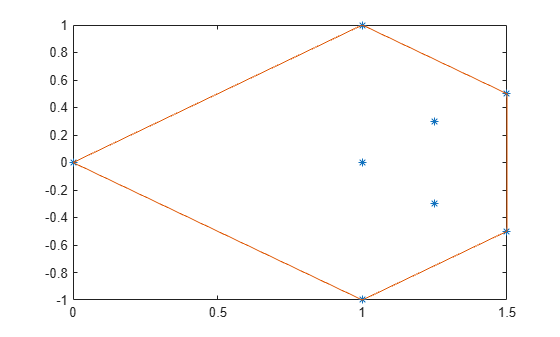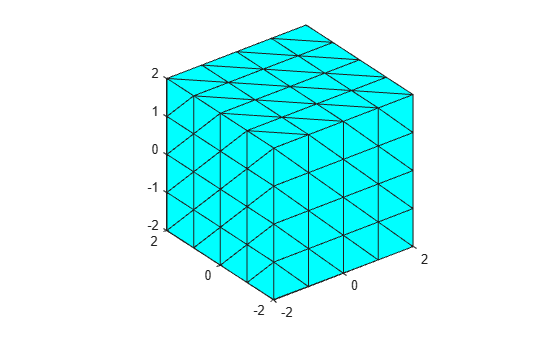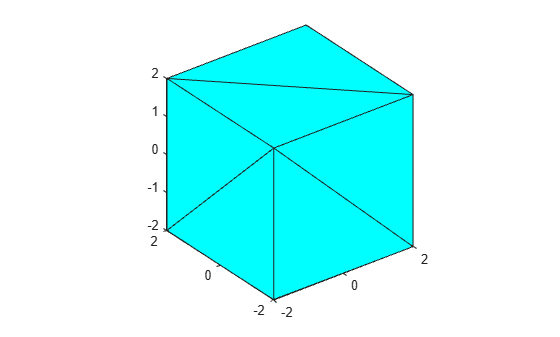convhull

Description

example

k = convhull(P) computes the 2-D or 3-D convex hull of the points in matrix P.

k = convhull(x,y) computes the 2-D convex hull of the points in column vectors x and y.

example

k = convhull(x,y,z) computes the 3-D convex hull of the points in column vectors x, y, and z.

example

k = convhull(___,'Simplify',tf) specifies whether to remove vertices that do not contribute to the area or volume of the convex hull. tf is false by default.

example

[k,av] = convhull(___) also computes the area or volume of the convex hull.

Examples

collapse all

Create a matrix of 2-D points. Compute the convex hull and its area.

P = [0 0; 1 1; 1.5 0.5; 1.5 -0.5; 1.25 0.3; 1 0; 1.25 -0.3; 1 -1];
[k,av] = convhull(P);

Plot the original points and the convex hull.

plot(P(:,1),P(:,2),'*')
hold on
plot(P(k,1),P(k,2))Display the area.

av
av = 1.7500

Simplify a 3-D convex hull by removing points that do not affect its volume.

Create a set of 3-D points. Compute the convex hull and its volume. Plot the convex hull.

[x,y,z] = meshgrid(-2:1:2,-2:1:2,-2:1:2);
x = x(:);
y = y(:);
z = z(:);

[k1,av1] = convhull(x,y,z);

trisurf(k1,x,y,z,'FaceColor','cyan')
axis equalCompute and plot a simplified version of the convex hull that contains fewer points, but preserves the volume.

[k2,av2] = convhull(x,y,z,'Simplify',true);

trisurf(k2,x,y,z,'FaceColor','cyan')
axis equalDisplay the volumes of both convex hulls. The volumes are the same, but the simplified convex hull uses fewer points.

av1
av1 = 64.0000
av2
av2 = 64

Input Arguments

collapse all

Points, specified as a matrix whose columns are the x-coordinates, y-coordinates, and (in three dimensions) z-coordinates.

x-coordinates, specified as a column vector.

y-coordinates, specified as a column vector.

z-coordinates, specified as a column vector.

Simplify indicator, specified as a numeric or logical 0 (false) or 1 (true).

Output Arguments

collapse all

Indices, returned as a vector or matrix.

• For 2-D points, k is a column vector containing the row indices of the input points that make up the convex hull, arranged counterclockwise.

• For 3-D points, k is a 3-column matrix representing a triangulation that makes up the convex hull. Each row represents a facet of the triangulation. The values represent the row indices of the input points.

Area or volume of the convex hull, returned as a scalar.

Tips

• To plot the output of convhull in two dimensions, use the plot function. To plot the output of convhull in three dimensions, use trisurf or trimesh.